Most Affordable JEE | NEET | 8,9,10 Preparation by Kota's Top IITian Doctor Faculties

# NCERT Solutions for Class 9 Maths chapter 8 Exercise 8.1 - Quadrilaterals

Class 9Hey, are you a class 9 Student and Looking for Ways to Download NCERT Solutions for Class 9 Maths chapter 8 Exercise 8.1? If Yes then you are at the right place.

Here we have listed Class 9 maths chapter 8 exercise 8.1 solutions in PDF that is prepared by Kota’s top IITian’s Faculties by keeping Simplicity in mind.

If you want to score high in your class 9 Maths Exam then it is very important for you to have a good knowledge of all the important topics, so to learn and practice those topics you can use eSaral NCERT Solutions.

So, without wasting more time Let’s start.

### Download The PDF of NCERT Solutions for Class 9 Maths chapter 8 Exercise 8.1 "Quadrilaterals"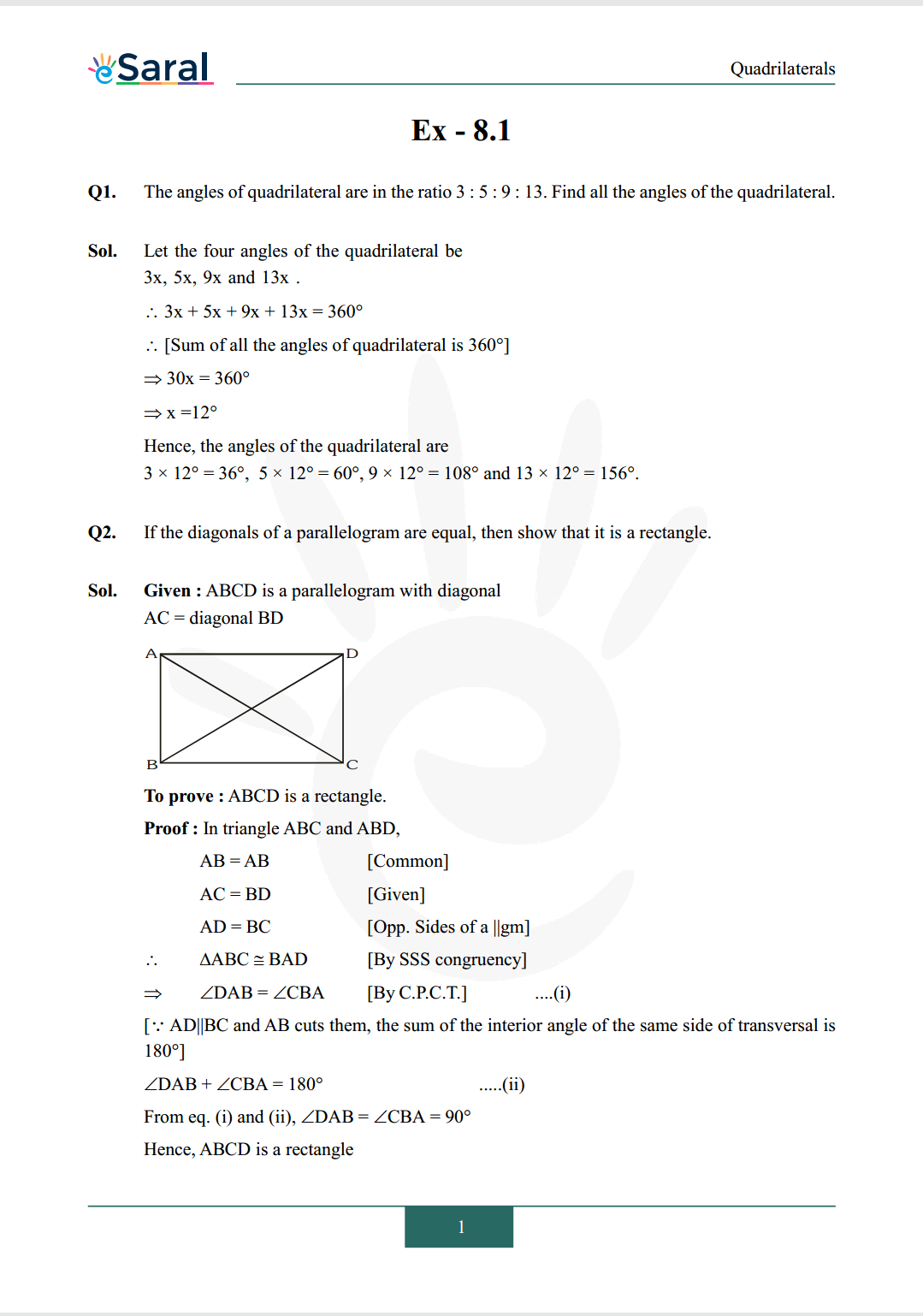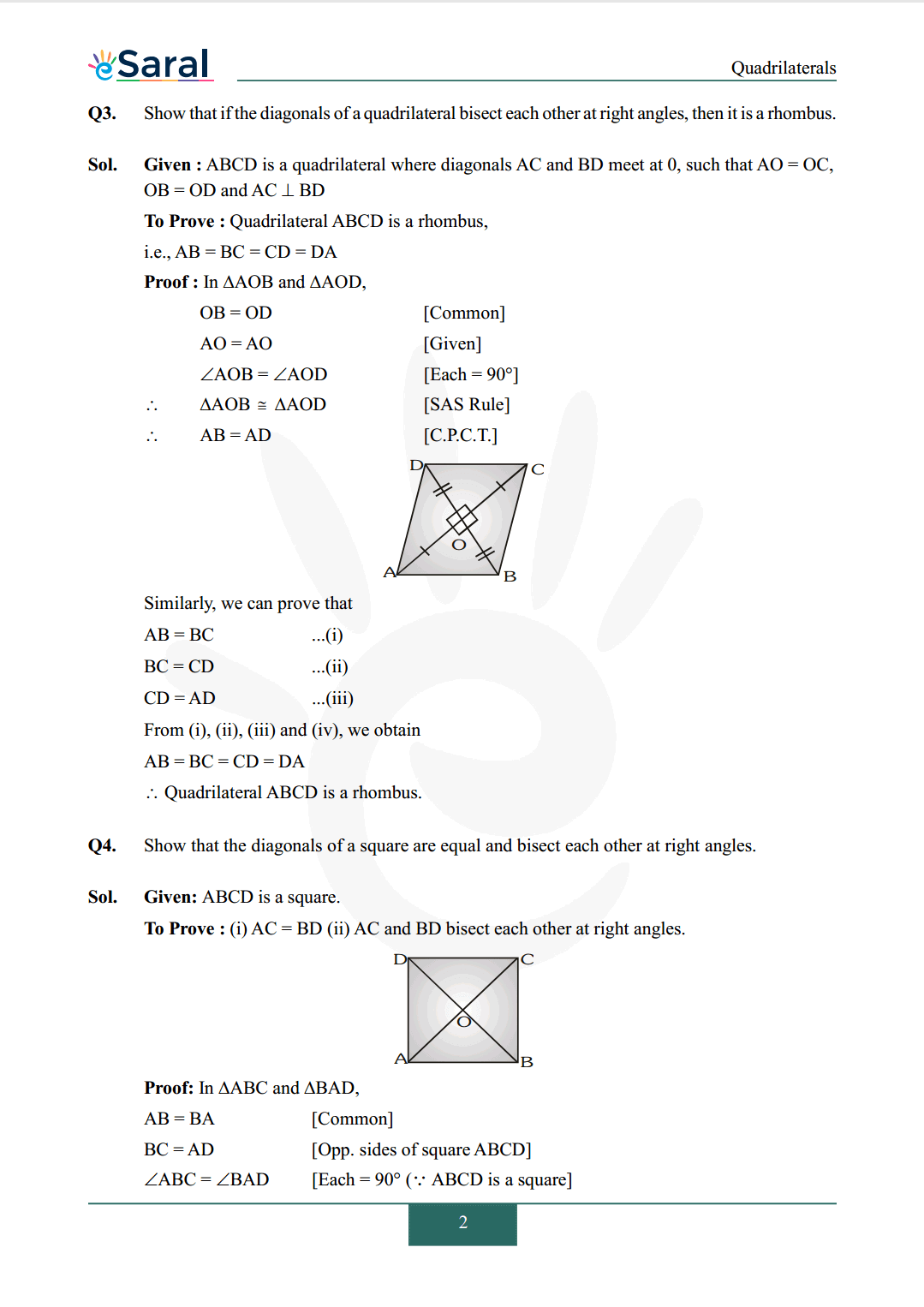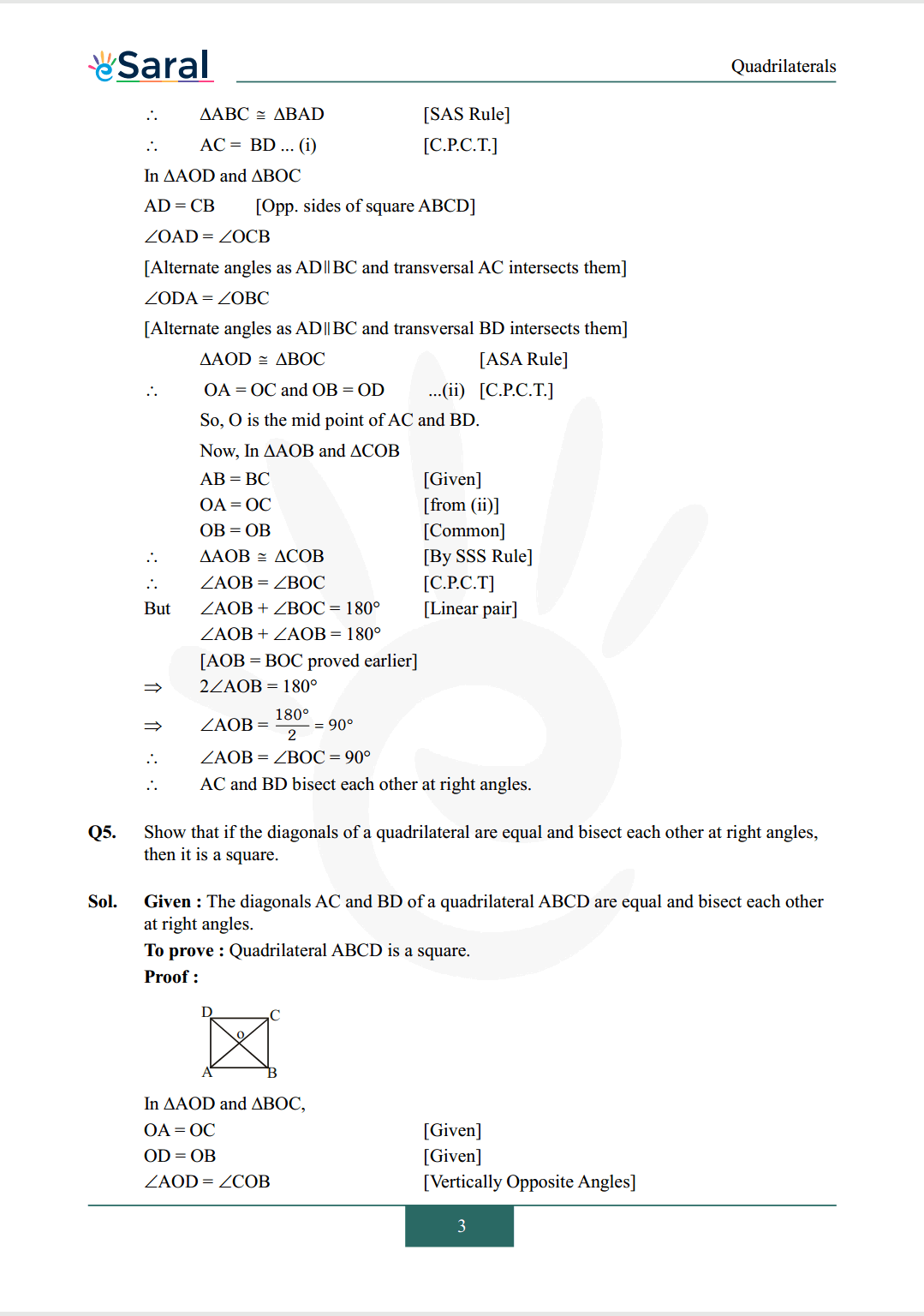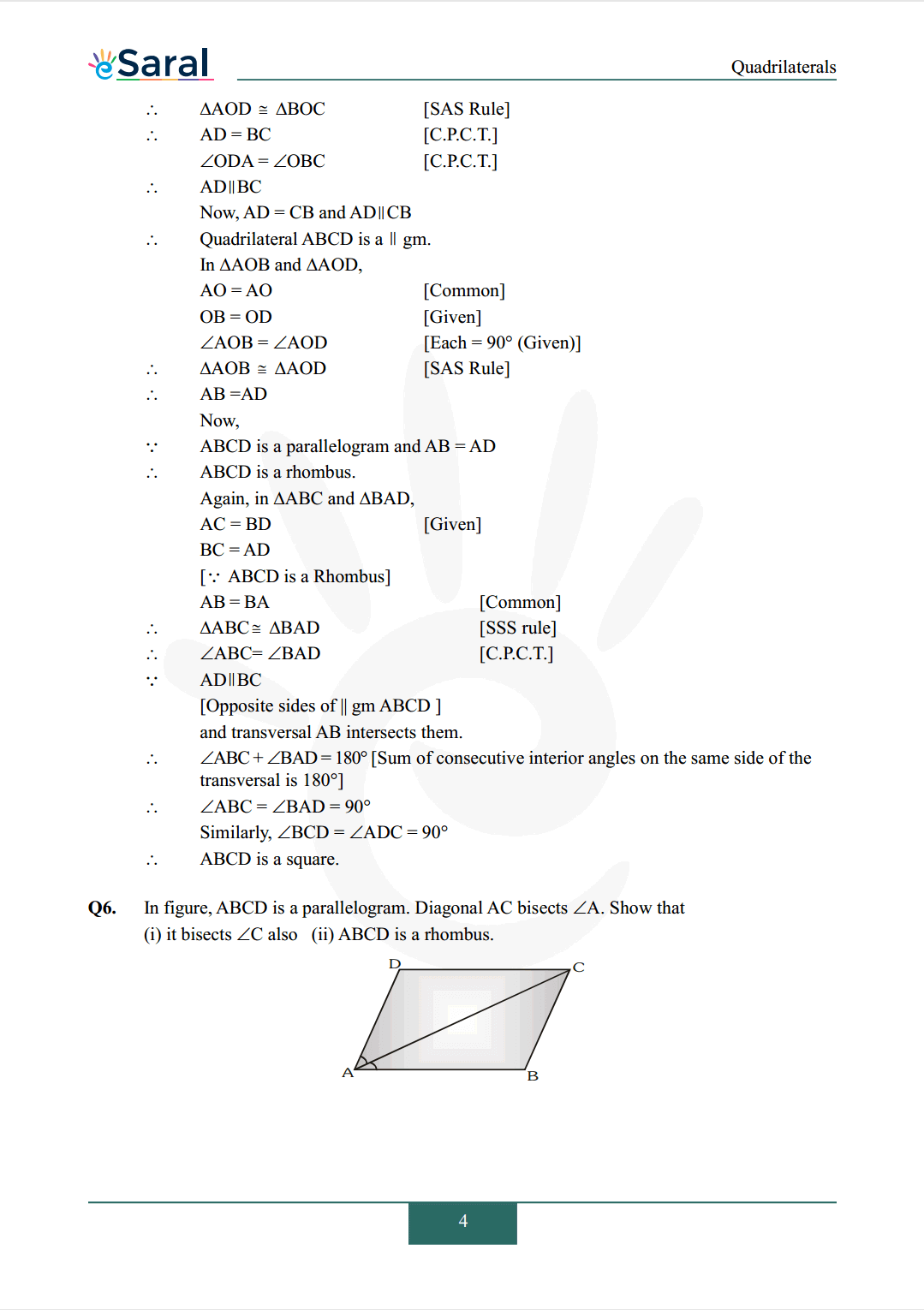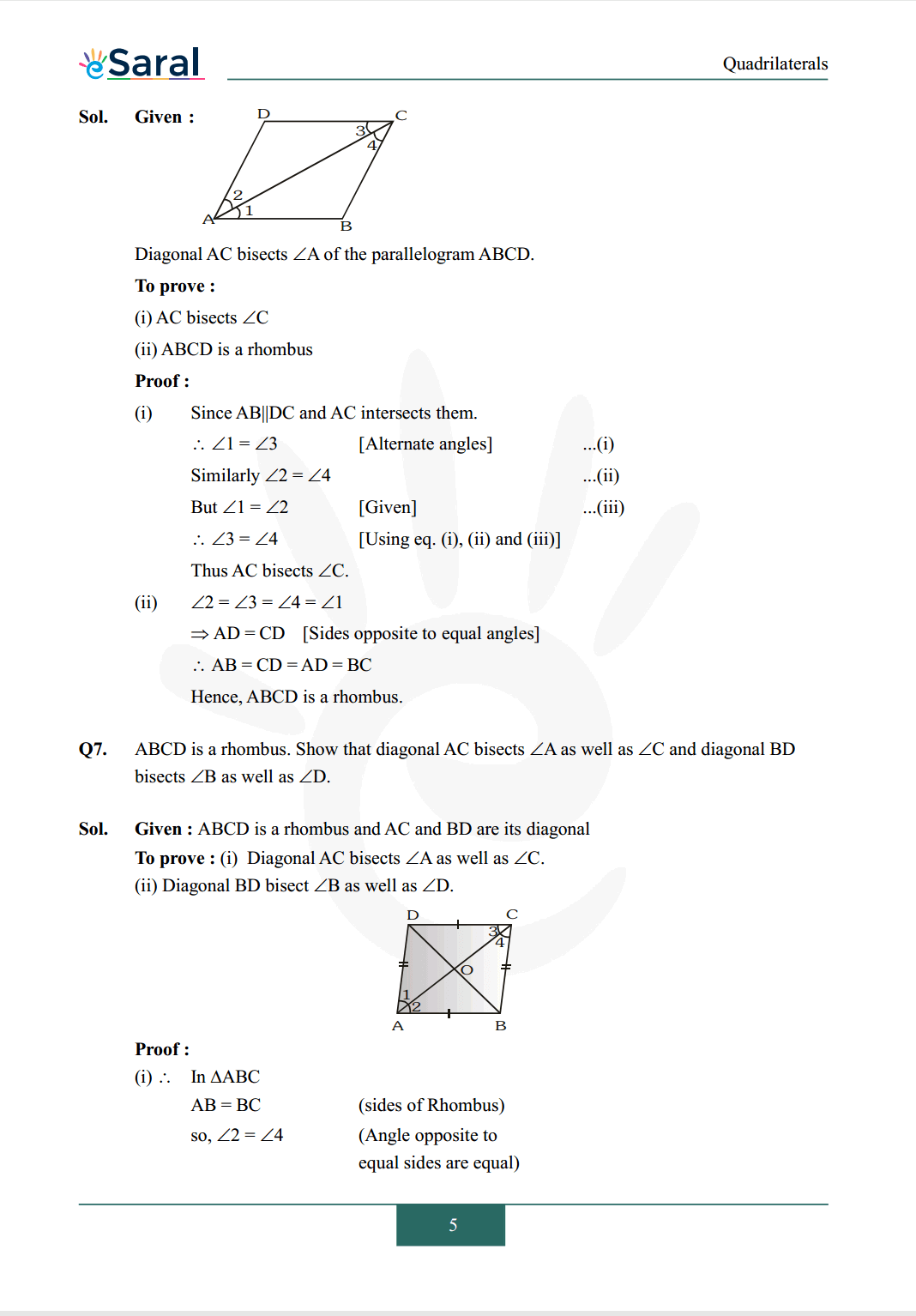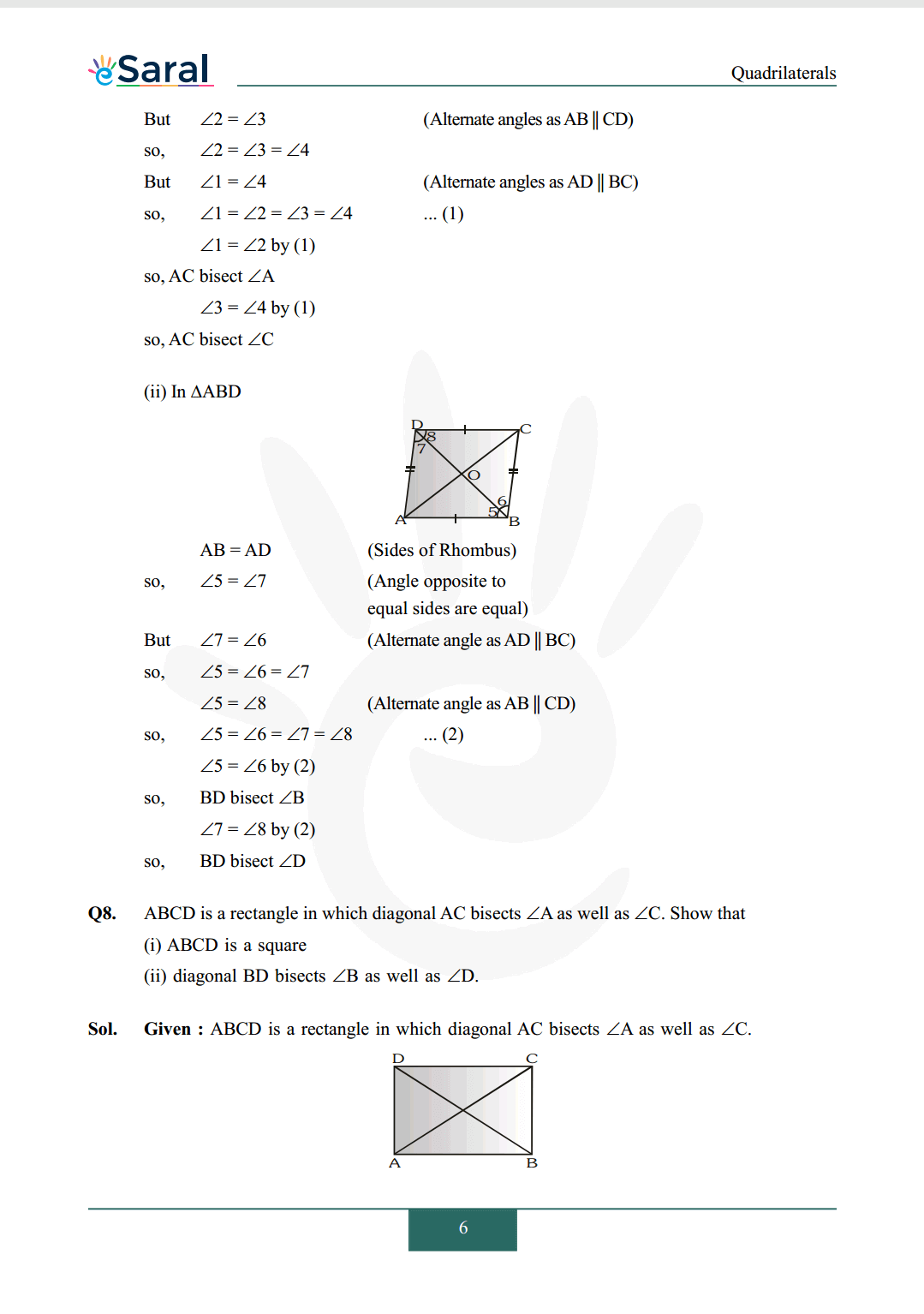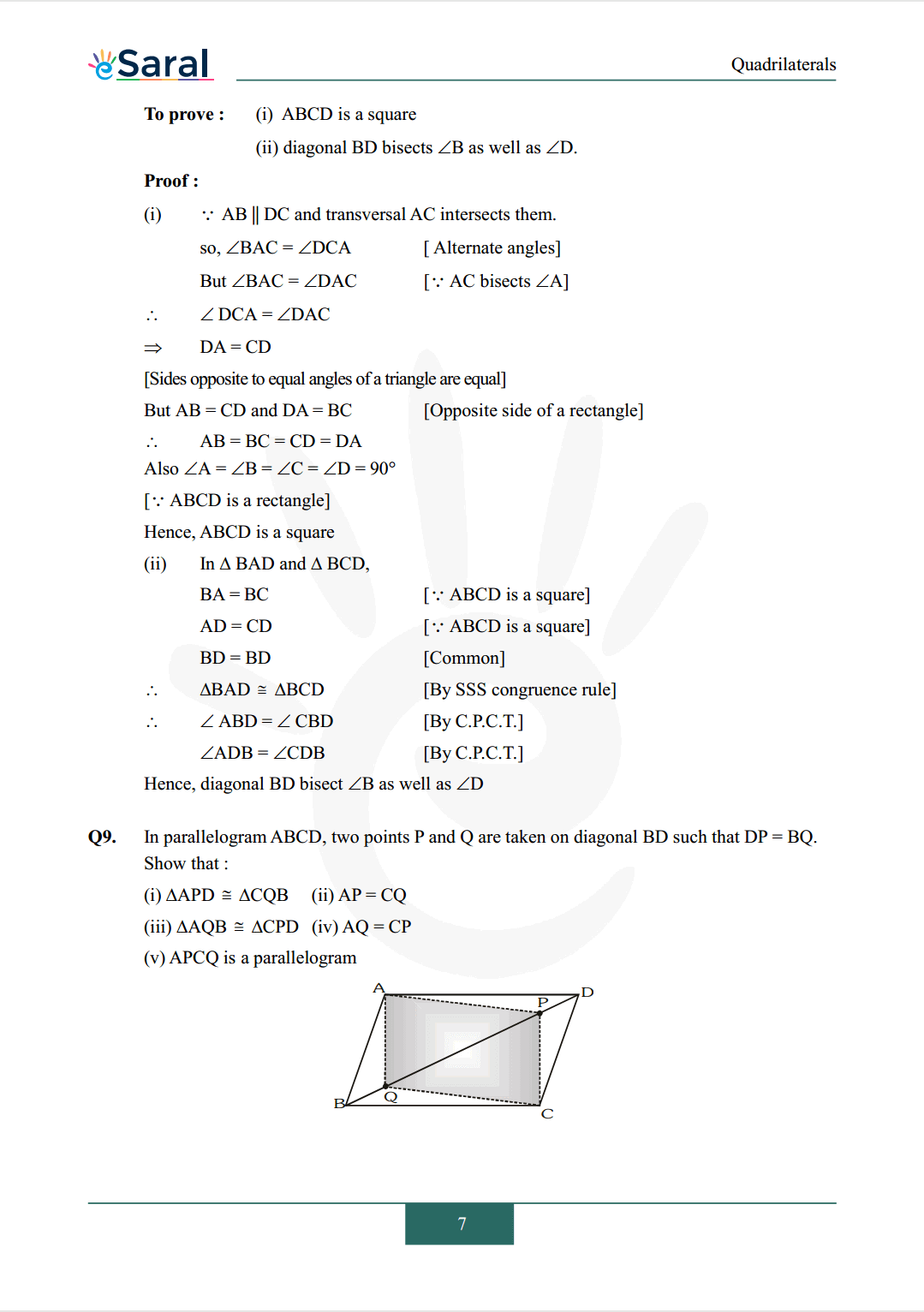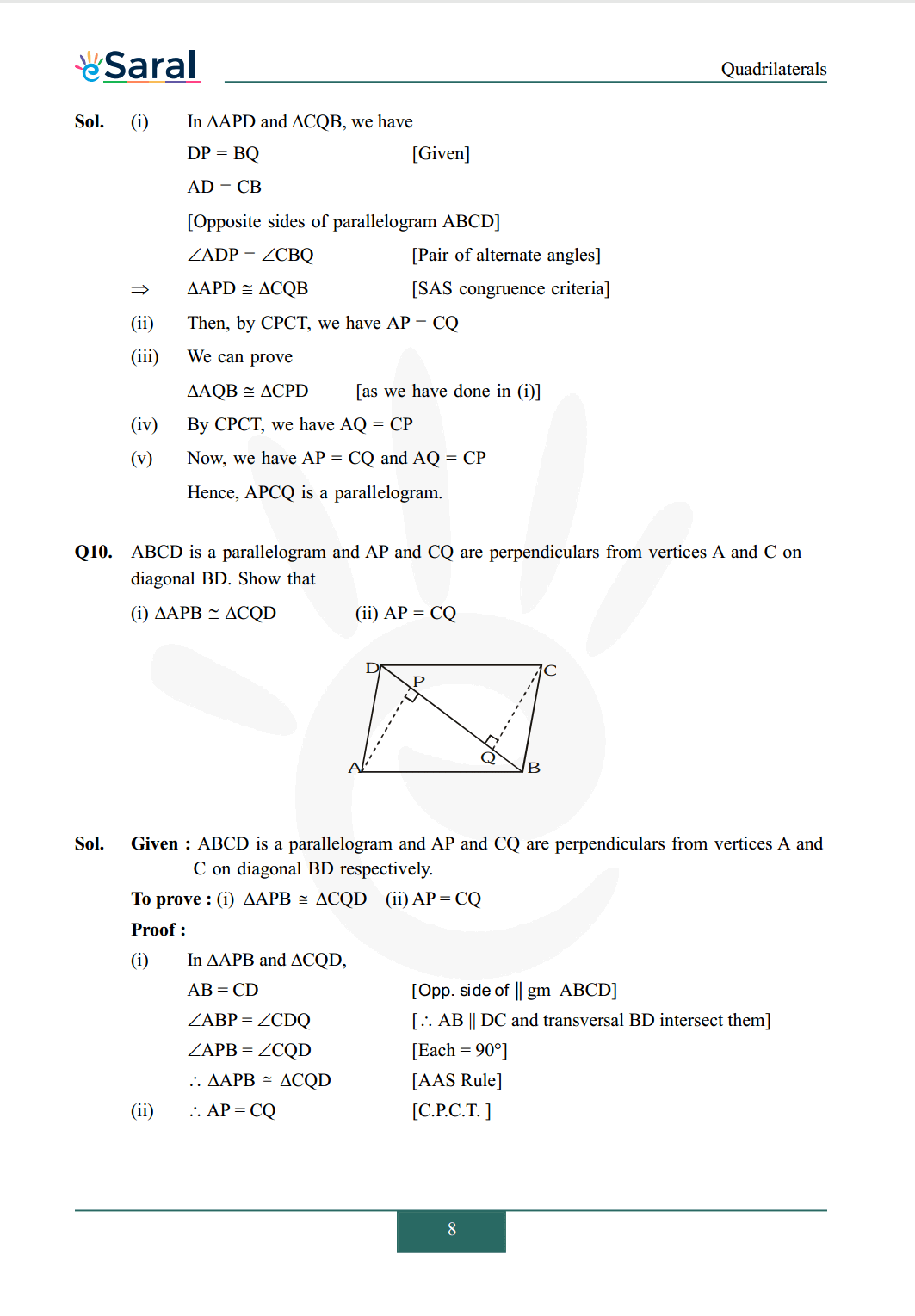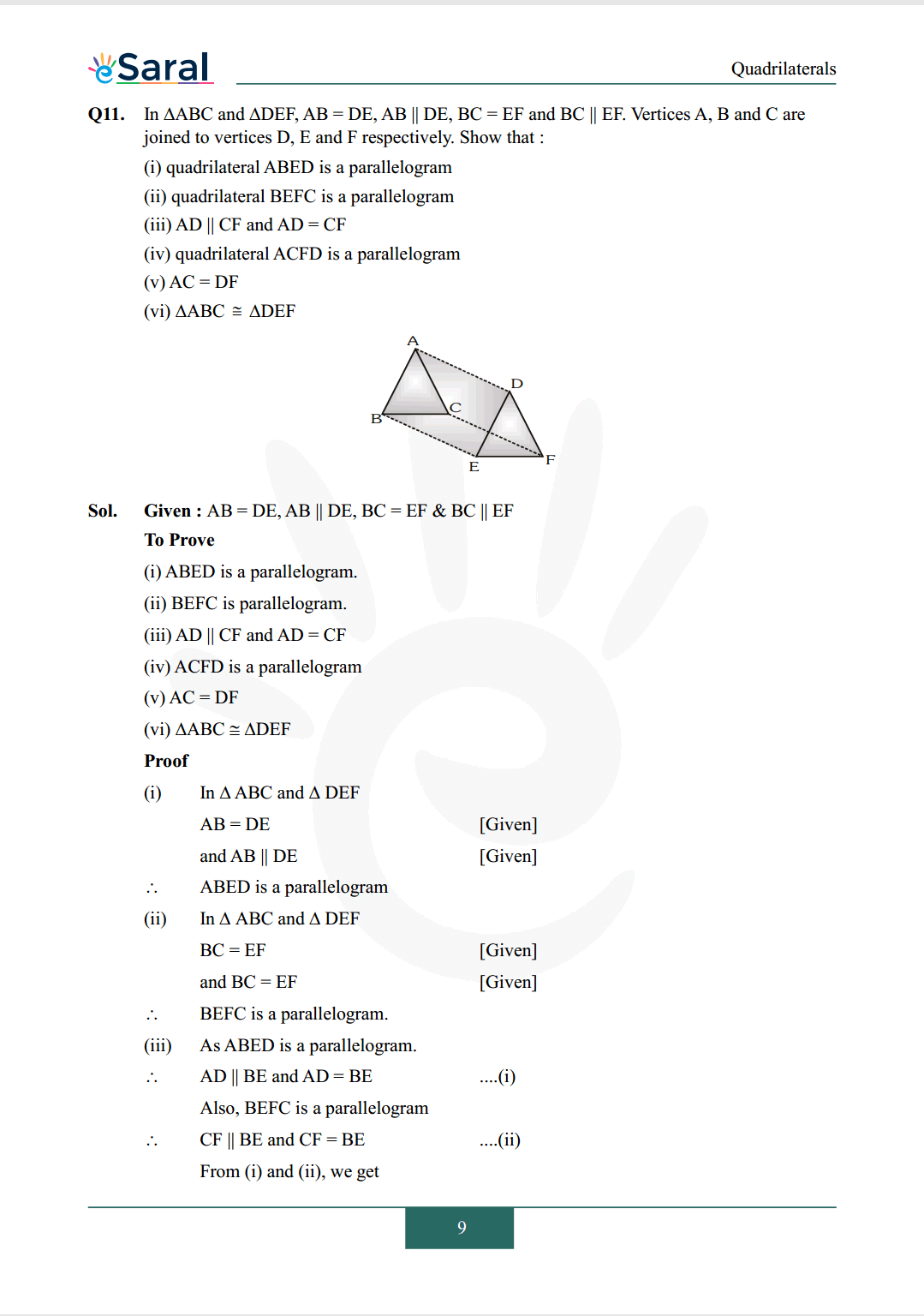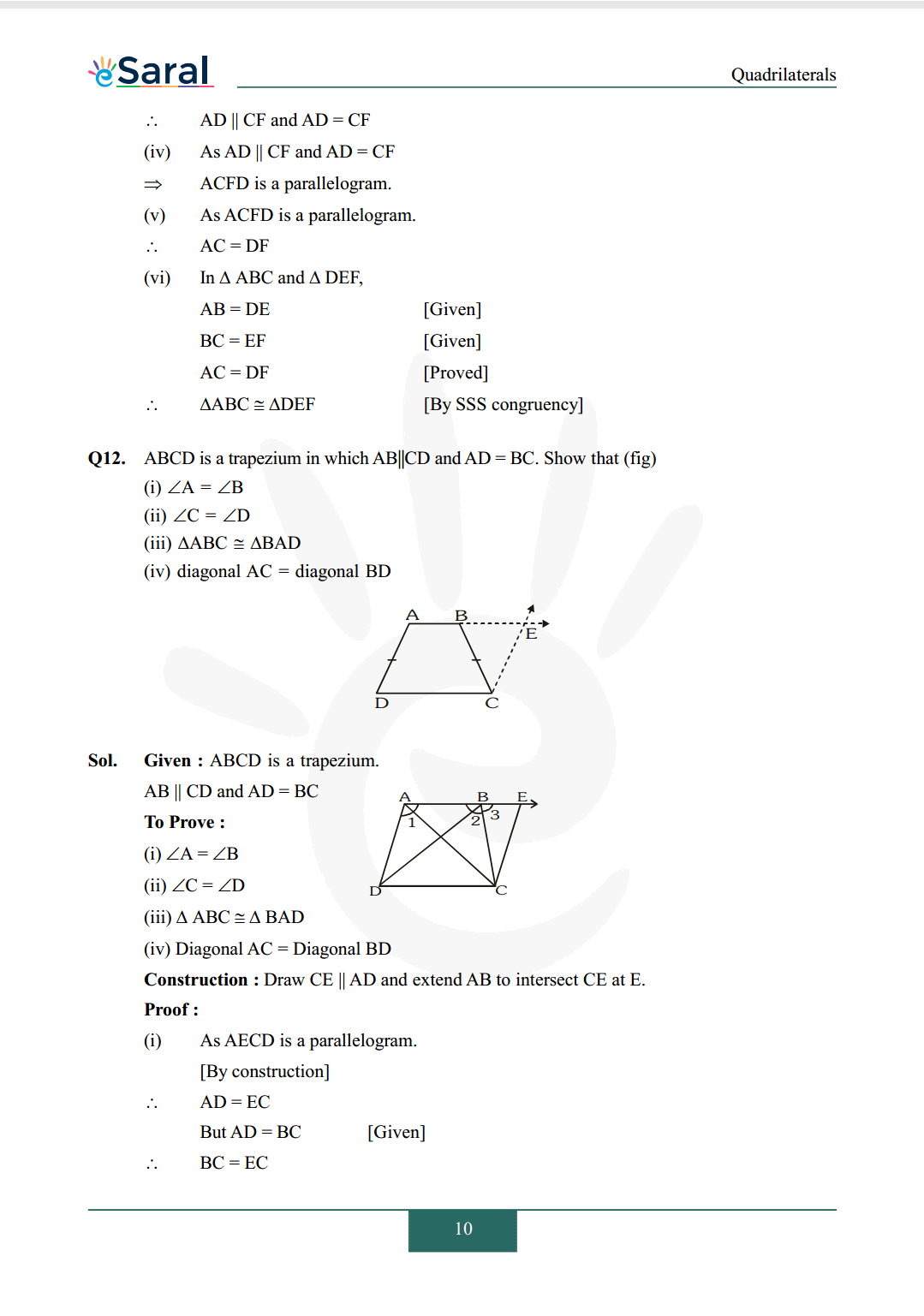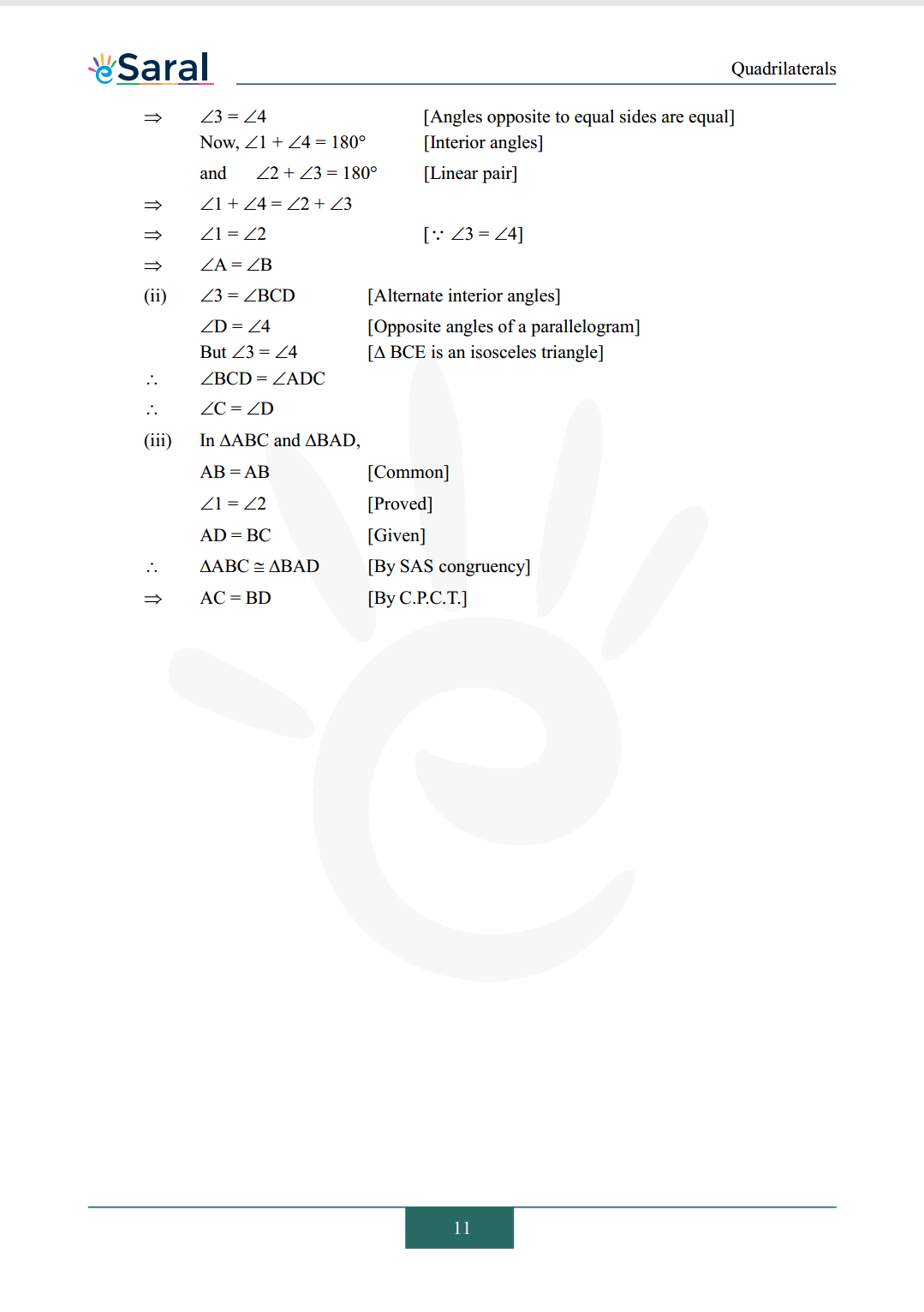#### All Questions of Chapter 8 Exercise 8.1

Once you complete the chapter 8 then you can revise Ex. 8.1 by solving following questions

Q1. The angles of quadrilateral are in the ratio $3: 5: 9: 13$. Find all the angles of the quadrilateral.

Q2. If the diagonals of a parallelogram are equal, then show that it is a rectangle.

Q3. Show that if the diagonals of a quadrilateral bisect each other at right angles, then it is a rhombus.

Q4. Show that the diagonals of a square are equal and bisect each other at right angles.

Q5. Show that if the diagonals of a quadrilateral are equal and bisect each other at right angles, then it is a square.

Q6. In figure, $\mathrm{ABCD}$ is a parallelogram. Diagonal AC bisects $\angle \mathrm{A}$. Show that
(i) it bisects $\angle \mathrm{C}$ also
(ii) $\mathrm{ABCD}$ is a rhombus.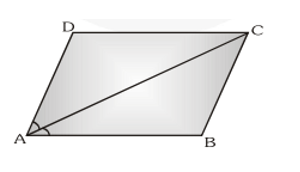Q7. $\mathrm{ABCD}$ is a rhombus. Show that diagonal $\mathrm{AC}$ bisects $\angle \mathrm{A}$ as well as $\angle \mathrm{C}$ and diagonal $\mathrm{BD}$ bisects $\angle \mathrm{B}$ as well as $\angle \mathrm{D}$.

Q8. $\mathrm{ABCD}$ is a rectangle in which diagonal AC bisects $\angle \mathrm{A}$ as well as $\angle \mathrm{C}$. Show that
(i) $\mathrm{ABCD}$ is a square
(ii) diagonal BD bisects $\angle \mathrm{B}$ as well as $\angle \mathrm{D}$.

Q9. In parallelogram $\mathrm{ABCD}$, two points $\mathrm{P}$ and $\mathrm{Q}$ are taken on diagonal $\mathrm{BD}$ such that $\mathrm{DP}=\mathrm{BQ}$. Show that :
(i) $\triangle \mathrm{APD} \cong \Delta \mathrm{CQB}$
(ii) $\mathrm{AP}=\mathrm{CQ}$
(iii) $\triangle \mathrm{AQB} \cong \Delta \mathrm{CPD}$
(iv) $\mathrm{AQ}=\mathrm{CP}$
(v) APCQ is a parallelogram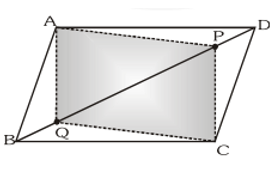Q10. $\mathrm{ABCD}$ is a parallelogram and $\mathrm{AP}$ and $\mathrm{CQ}$ are perpendiculars from vertices $\mathrm{A}$ and $\mathrm{C}$ on diagonal BD. Show that
(i) $\triangle \mathrm{APB} \cong \Delta \mathrm{CQD}$
(ii) $\mathrm{AP}=\mathrm{CQ}$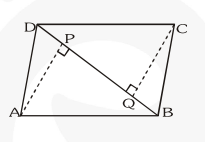Q11. In $\triangle \mathrm{ABC}$ and $\triangle \mathrm{DEF}, \mathrm{AB}=\mathrm{DE}, \mathrm{AB} \| \mathrm{DE}, \mathrm{BC}=\mathrm{EF}$ and $\mathrm{BC} \| \mathrm{EF}$. Vertices $\mathrm{A}, \mathrm{B}$ and $\mathrm{C}$ are joined to vertices $\mathrm{D}, \mathrm{E}$ and $\mathrm{F}$ respectively. Show that :
(i) quadrilateral ABED is a parallelogram
(ii) quadrilateral BEFC is a parallelogram
(iii) $\mathrm{AD} \| \mathrm{CF}$ and $\mathrm{AD}=\mathrm{CF}$
(iv) quadrilateral ACFD is a parallelogram
(v) $\mathrm{AC}=\mathrm{DF}$
(vi) $\triangle \mathrm{ABC} \cong \Delta \mathrm{DEF}$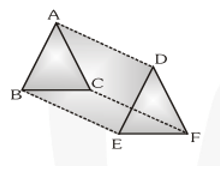Q12. $\mathrm{ABCD}$ is a trapezium in which $\mathrm{AB} \| \mathrm{CD}$ and $\mathrm{AD}=\mathrm{BC}$. Show that (fig)
(i) $\angle \mathrm{A}=\angle \mathrm{B}$
(ii) $\angle \mathrm{C}=\angle \mathrm{D}$
(iii) $\Delta \mathrm{ABC} \cong \Delta \mathrm{BAD}$
(iv) diagonal $\mathrm{AC}=$ diagonal $\mathrm{BD}$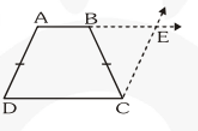Class 9 NCERT Maths Book PDF

Class 9 NCERT Maths Exemplar PDF

Complete Solutions for Class 9 Maths chapter 8

Class 9 Maths Chapter 7 Exercise 7.1 Solutions Free

Class 9 Maths Chapter 7 Exercise 7.2 Solutions Free

Class 9 Maths Chapter 7 Exercise 7.3 Solutions Free

Class 9 Maths Chapter 7 Exercise 7.4 Solutions Free

If you have any Confusion related to NCERT Solutions for Class 9 Maths chapter 8 Exercise 8.1 then feel free to ask in the comments section down below.

To watch Free Learning Videos on Class 9 by Kota’s top Faculties Install the eSaral App